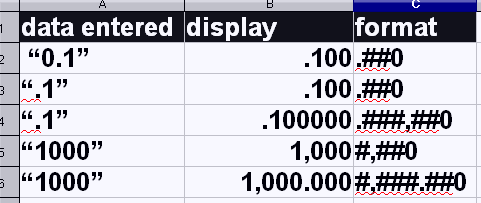# (Calc) user-defined number format: how to limit number of digits displayed to column width?

Hello,

Title says it all. I’ve set up a user-defined number format to use as default, but every now and then I get a result that is longer than what fits inside the cell, and then Calc only displays ###. I’ve noticed that the General number format doesn’t have this problem as there the number is truncated and rounded so that it fits inside the cell, no matter the width.

Is there a way to enable this behavior for user-defined formats? I’ve tried looking here and on the wiki, but haven’t found anything relevant, then again, it seems not all of the formatting options are documented.

-Lou

PS: I should probably add this—even if there’s no fix for my problem above, you might be able to help me with the underlying issue.

The reason why I have created the user-defined number format in the first place is that I want to enable the thousands separator and drop the leading zero. These are my regional defaults as well (US,) but Calc still displays 0.1 and 1000, instead of .1 and 1,000 for example.

Maybe there’s a way to make the General format respect these settings?

I’m on Win7 Home Premium x64 SP1.

Do you mean Shrink to fit cell size ?

@JohnSUN: No, not really. What that option does is decrease the font size (often to too small.) The default behavior for the General format is to truncate the digits that do not fit inside the cell and round the rest of the number. This is what I’d like to reproduce with a custom number format.

Yes, now I knew… I was post this comment before you add your second message

Try this issue - Format cells with engineering notation

@JohnSUN: Thanks, but my issue is not specifically a formatting one: I’m happy with the number format I’ve set up. My problem is related to how it is displayed in Calc, if an input or result exceeds the column width limit. Furthermore, I’ve looked at some of the examples in the linked discussion, and I’ve seen the same ### representation with long numbers that I’d like to get rid of.

@ROSt53: Thanks, but this is not what I’m looking for.

@Mark12547: Thank you for understanding my problem. I’d hoped there would be a way to apply these IF conditions to input fields somehow. I guess the corresponding behavior of the General format is hard-coded and it cannot be achieved with user-defined ones. Oh well.

I following this thread already for a few days but I am not sure if I understand the question correct. If I understand the problem correct, below could be the solution.

In Calc you can create any format you need to have. Based on the original question with “.1” and “1,000”

I created some examples:And here is where you can enter the format “code”. Just type the code into the field indicated. The “code” examples are above.

If you like to know more about data format “codes” search quickly the web. you should find all information you need.

Whenever you see “###” your column width is to small. You can either:

• increase the column width
• reduce the font size (see comment of @JohnSUN)
• reduce number of digits after the decimal point
Which one you use depends on your needs and constraints

If this is an input field (i.e., where someone would be typing values), the best I can suggest is to put only two #'s behind the decimal point in your user-defined format code, e.g., `#,###.##`

The disadvantage of this is that you would see at most only two digits after the decimal point, so a number like 0.001 will display as . (just a single period).

If this is for display only, but not for entering values, one could calculate the value somewhere else and then use a formula consisting of an IF to range-check the number and the resulting value be TEXT to convert as per one’s chosen formula, e.g., cell A2 could hold a formula like:

``````=TEXT(A1,IF(OR(A1>=10000, A1<=-10000),"#,###.##","#,###.#######"))
``````

The IF would be true if the number (in cell A1) is over 10,000 or under -10,000 so the format used by TEXT would be “#,###.##”, but if the number is within the -10,000 to +10,000 (minus the end points), the format used by TEXT would be “#,###.######”. If you want to have the text right-justified or center, you would have to use “Format cell” → “alignment” tab to set horizontal alignment to right or center.

If you want to implement more masks based on more formatting ranges, the IF part would get more complicated, e.g.,

``````=TEXT(A1,IF(OR(A1>=1000000,A1<1000000),"#,###",
IF(OR(A1>=10000 , A1<=-10000),"#,###.##","#,###.#######")))
``````

(The above formula split into multiple lines for clarity; it would be entered into the cell as one long line.)

Of course, you would have to adjust the masks and ranges to what are reasonable values for your application and the width of the display field.